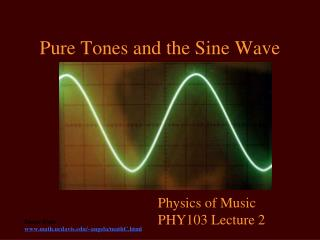DownloadDownload PresentationPure Tones and the Sine Wave

# Pure Tones and the Sine Wave

Télécharger la présentation## Pure Tones and the Sine Wave

- - - - - - - - - - - - - - - - - - - - - - - - - - - E N D - - - - - - - - - - - - - - - - - - - - - - - - - - -
##### Presentation Transcript

1. Pure Tones and the Sine Wave Physics of Music PHY103 Lecture 2 Image from www.math.ucdavis.edu/~angela/mathC.html

2. Trig definition of a sine wave Period or wavelength amplitude From math learning service U. Adelaide

3. Harmonic motion From ecommons.uwinnipeg.ca/ archive/00000030/

4. Velocity and PositionSine and Cosine From www2.scc-fl.com/lvosbury/AnimationsForTrigono... Sine and Cosine

5. Pythagorean theoremConservation of Energy When the spring is extended, the velocity is zero. When the spring is in the middle, the velocity is maximum. The position is the sine wave, the velocity is the cosine wave. Kinetic energy (square of velocity) + Potential energy (square of position) is total energy is conserved. b=c Sin(θ) θ a=c Cos(θ)

6. Making a pure tone with Matlab

7. Period (units time or seconds) Sine Wave Amplitude

8. Wavelength (units cm) Sine Wave Amplitude Position x For a wave on water or on a string - spatial variation instead of temporal variation

9. Amplitude • Units depend on what is measured • Velocity, pressure, voltage? Angular frequency angular frequency radians per second frequency in Hz cycles per second

10. Relation between frequency and period • Suppose the period is P=0.2s • I need 5 cycles to add up to 1s • So the frequency is f=5Hz. • The number of periods/cycles that add up to 1 second is the frequency fP=1f=1/P

11. Relation between frequency and period P=1/f 1 second 12 cycles in 1 second The frequency is 12 Hz The period (time between each peak is 1/12 seconds or 0.083seconds

12. How does energy/power depend on the amplitude? • Energy depends on the sum of the square of velocity and square of position (from equilibrium) • We expect that the energy or power (energy per second for a traveling wave) depends on the square of the amplitude.

13. Decay – loss of energy

14. Showing a sine wave on the oscilloscope

15. Signal or waveform generator Can adjust • Shape of wave (sine, triangle, square wave) • Voltage (amplitude of wave) • Frequency of wave Oscilloscope Adjust voltage of display (y–axis) Adjust time shown in display (x-axis) Adjust trigger Can also place display in x-y mode so can generator Lissajous figures

16. Sine waves – one amplitude/ one frequency Sounds as a series of pressure or motion variations in air. Sounds as a sum of different signals each with a different frequency.

17. Clarinet spectrum Clarinet spectrum with only the lowest harmonic remaining Frequency Time 

18. Waveform viewFull sound Only lowest harmonic Complex tone Pure tone

19. Decomposition into sine waves • We can look at a sound in terms of its pressure variations as a function of time OR • We can look at a sound in terms of its frequency spectrum This is equivalent to saying each segment is equivalent to a sum of sine waves. “Fourier decomposition” Some of the character or “timbre” of different sounds comes from its spectrum: which harmonics are present, how strong they are, and where they are exactly (they can be shifted from integer ratios)

20. Audition tutorial:Pulling up the spectral view

21. Zoom in vertical axis Record Zoom in horizontal axis Loop Play

22. Right click and hold on the axes will also allow you to adjust the range

23. Getting a linear frequency spectrum

24. Traveling waves

25. Traveling waves • Right traveling • Left traveling Law of cosines

26. Why the second mode has twice the frequency of the fundamental • Exciting the fundamental. Excite a pulse and then wait until it goes down the string and comes all the way back. • Exciting the second harmonic. When the first pulse gets to the end the string, you excite the next pulse. This means you excite pulses twice as often. You must drive at twice the frequency to excite the second mode

27. Traveling waves vs standing waves • Can think of standing waves as sums of left traveling and right traveling waves • The time to go from zero to max depends on the time for the wave to travel a distance of 1 wavelength smaller wavelengths have faster oscillation periods (frequencies) • If the waves move faster on the string then the modes of oscillation (the standing waves) will be higher frequency

28. Wave speed dimensional analysis • Only quantities we have available: • String density (mass per unit length) ρ • String length L • Tension on string T • Force = mass times acceleration F=ma (units g cm/s2) • Tension on a string is set by the force pulling on the string So T is units of g cm/s2 mg

29. Wave speed dimensional analysis continued • We want a velocity (cm/s). How do we combine the 3 physical quantities to get a velocity? • String density ρ(g/cm) • String length L (cm) • Tension T (g cm/s2) • T/ ρ has units cm2/s2 so a velocity is given by • To get a quantity in units of frequency we divide a velocity by a length • When we think about oscillating solids (copper pipes for example) the thickness is also important.

30. Terms covered • Harmonic motion • Oscilloscope • Wave generator or signal generator • Pure tone • Complex tone • Spectrum • Timbre • Amplitude • Angular frequency • Frequency • Hz (Hertz) • Energy • Period • Wavelength

31. Recommended reading • Berg and Stork Chap 1 on Harmonic Motion